Test: Transmission Lines- 2

# Test: Transmission Lines- 2

Test Description

## 20 Questions MCQ Test GATE Electrical Engineering (EE) 2023 Mock Test Series | Test: Transmission Lines- 2

Test: Transmission Lines- 2 for Electronics and Communication Engineering (ECE) 2023 is part of GATE Electrical Engineering (EE) 2023 Mock Test Series preparation. The Test: Transmission Lines- 2 questions and answers have been prepared according to the Electronics and Communication Engineering (ECE) exam syllabus.The Test: Transmission Lines- 2 MCQs are made for Electronics and Communication Engineering (ECE) 2023 Exam. Find important definitions, questions, notes, meanings, examples, exercises, MCQs and online tests for Test: Transmission Lines- 2 below.
Solutions of Test: Transmission Lines- 2 questions in English are available as part of our GATE Electrical Engineering (EE) 2023 Mock Test Series for Electronics and Communication Engineering (ECE) & Test: Transmission Lines- 2 solutions in Hindi for GATE Electrical Engineering (EE) 2023 Mock Test Series course. Download more important topics, notes, lectures and mock test series for Electronics and Communication Engineering (ECE) Exam by signing up for free. Attempt Test: Transmission Lines- 2 | 20 questions in 60 minutes | Mock test for Electronics and Communication Engineering (ECE) preparation | Free important questions MCQ to study GATE Electrical Engineering (EE) 2023 Mock Test Series for Electronics and Communication Engineering (ECE) Exam | Download free PDF with solutions
 1 Crore+ students have signed up on EduRev. Have you?
Test: Transmission Lines- 2 - Question 1

### Double stub matching eliminates standing waves on the

Detailed Solution for Test: Transmission Lines- 2 - Question 1

Double stub matching eliminates standing waves on the source side of the left stub.

Test: Transmission Lines- 2 - Question 2

### If Zsc = 64Ω and Zoc = 100 Ω, the characteristic impedance will be given by.

Detailed Solution for Test: Transmission Lines- 2 - Question 2

Characteristic impedance is given by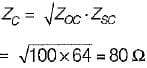Test: Transmission Lines- 2 - Question 3

### Consider a lossless line with characteristic impedance R0 and VSWR = S. Then, the impedance at the point of voltage maxima and voltage minima are respectively given by

Detailed Solution for Test: Transmission Lines- 2 - Question 3

Given,
Z0 = R0
we have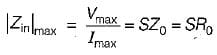and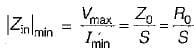Test: Transmission Lines- 2 - Question 4

If K is the reflection coefficient and S is the voltage standing wave ratio, then

Detailed Solution for Test: Transmission Lines- 2 - Question 4

Voltage standing wave ratio is given by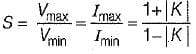or,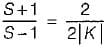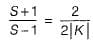(Using componendo and dividendo)
or,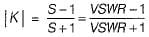Test: Transmission Lines- 2 - Question 5

In terms of R, L, G and C the propagation constant of a transmission line is given by

Detailed Solution for Test: Transmission Lines- 2 - Question 5

Propagation constant of a transmission line is given by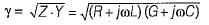Test: Transmission Lines- 2 - Question 6

A line has  Z0 = 300∠0º Ω, and ZL = 150∠0º Ω  Voltage standing wave ratio (S) is given by

Detailed Solution for Test: Transmission Lines- 2 - Question 6

Given
Z0 = 300∠0º
and ZL =150∠0ºΩ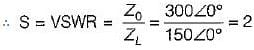Test: Transmission Lines- 2 - Question 7

The characteristic impedance of a distortionless line is

Detailed Solution for Test: Transmission Lines- 2 - Question 7

Characteristic impedance,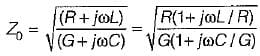or,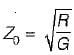(Since L/R = C/G for a distortionless line)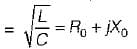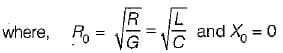Hence, characteristic impedance of a distortionless line is purely real.

Test: Transmission Lines- 2 - Question 8

A transmission line works as a

Test: Transmission Lines- 2 - Question 9

A transmission line with a characteristic impedance Z0 is connected to a transmission line with characteristic impedance Zc. if the systme is being driven by a generator connected to the first line, then the overall transmission coefficient of current will be

Detailed Solution for Test: Transmission Lines- 2 - Question 9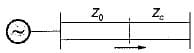The overall transmission coefficient is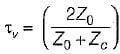Test: Transmission Lines- 2 - Question 10

Which of the following statements related to a transmission line is/are correct?
1. Transmission line elements are integral parts of the antenna, in some antenna system.
2. A feeder is a particular case of a transmission Sine.
3. A lossless transmission line doesn’t has resistance but, has a non-zero value of leakage conductance.
4. At radio frequency (RF), R and G both are neglected.

Detailed Solution for Test: Transmission Lines- 2 - Question 10
• Statements-1 and 2 are true.
• Statement-3 is not correct because in a lossless transmission line there is neither resistive loss (i.e. R = 0) nor any leakage current (i.e. G = 0).
• At radio frequency (RF), the inductive reactance is much larger than the resistance and so also the capacitie susceptance in comparison to the shunt conductance. Hence, at radio frequencies R and G both are neglected. Thus, statement-4 is correct.
Test: Transmission Lines- 2 - Question 11

Assertion (A): A finite transmission line terminated in its characteristic impedance Z0, is equivalent to an infinite line.
Reason (R): The input impedance of an infinite line is the characteristic impedance of the line.

Detailed Solution for Test: Transmission Lines- 2 - Question 11

The input impedance of a finite line terminated in its characteristic impedance (Z0), is equivalent to an infinite line because the input impedance of an infinite line is the characteristic impedance of the line (Z0). Hence, both assertion and reason are true and reason is the correct explanation of assertion.

Test: Transmission Lines- 2 - Question 12

Consider the following statements:
1. Propagation constant is a dimensionless quantity.
2. When the line is lossless, propagation constant is directly proportional to the frequency.
3. Propagation constant when multiplied with the frequency gives the electrical length of the line.
Which of the above statements is/are true?

Detailed Solution for Test: Transmission Lines- 2 - Question 12
• Propagation constant is dimensionless quantity because it is the ratio of voltages or currents.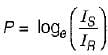or,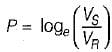(where, P = Propagation constant) Hence, statement-1 is correct.
• For a lossless transmission line,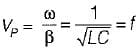∴ VP α f
Thus, statement - 2 is also correct.
• Statement-3 is also true.
Thus, all statements are true.
Test: Transmission Lines- 2 - Question 13

Assertion (A): The group velocity is usually more than the phase velocity.
Reason (R): If the transmission line or transmission medium is such that different frequencies travel with different velocities, then the line or the medium is said to be dispersive.

Detailed Solution for Test: Transmission Lines- 2 - Question 13

Group velocity is given by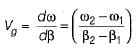Phase velocity or velocity of propagation is given by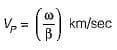The group velocity is usually less than the phase velocity. Hence, assertion is a false statement.

Test: Transmission Lines- 2 - Question 14

Assertion (A): A transmission line act as resonant circuit and is used in many applications at high frequencies in antenna design and other ratio circuitory
Reason (R): An open and short-circuited lines behaves like resonant circuit when length of line is an integral multiple of λ/3.

Detailed Solution for Test: Transmission Lines- 2 - Question 14

Assertion is correct because when a transmission line is open or short-circuited it behaves as resonant circuit. However, reason is false because this happens when length of the line is an integral multiple of λ/4.
We knnw that
Zoc = -j cot βl
and Zsc = jZ0 tan βl
Thus, when βl = length of line = nλ/4 , then
cot βl = 0
and tan βl = ∞
∴ Zoc = 0
and Zsc = ∞
This means a quarter wave short-circuit line represents an infinite impedance at inpul terminals, just like a parallel resonant (LC) circuil and a λ/4 open circuit line present zero impedance at input terminals just like a series resonant LC circuit.

Test: Transmission Lines- 2 - Question 15

Assertion (A): Sometimes a quarter wave line is called as impedance inverter.
Reason (R): The quarter wavelength line transforms a load impedance ZR that is smaller than Z0 into a value Zs that is larger than Z0 and vice-versa.

Test: Transmission Lines- 2 - Question 16

ln an impedance smith chart, a clockwise movement along a constant resistance circle gives rise to

Test: Transmission Lines- 2 - Question 17

A transmission line whose characteristic impedance is purely resistive

Test: Transmission Lines- 2 - Question 18

The input impedance of a short circuited quarter wave long transmission line is

Detailed Solution for Test: Transmission Lines- 2 - Question 18

Input impedance of a short-circuited quarter wave line depends on the nature of load impedance ZL.

Test: Transmission Lines- 2 - Question 19

A rectangular air filled waveguide has cross section of 4 cm x 10 cm. The minimum frequency which can propagate in the waveguide is

Detailed Solution for Test: Transmission Lines- 2 - Question 19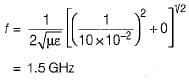Test: Transmission Lines- 2 - Question 20

Consider the transmission system shown below: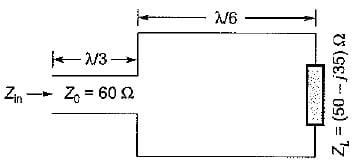The input impedance Zin is given by

## GATE Electrical Engineering (EE) 2023 Mock Test Series

22 docs|274 tests
 Use Code STAYHOME200 and get INR 200 additional OFF Use Coupon Code
Information about Test: Transmission Lines- 2 Page
In this test you can find the Exam questions for Test: Transmission Lines- 2 solved & explained in the simplest way possible. Besides giving Questions and answers for Test: Transmission Lines- 2, EduRev gives you an ample number of Online tests for practice

## GATE Electrical Engineering (EE) 2023 Mock Test Series

22 docs|274 tests